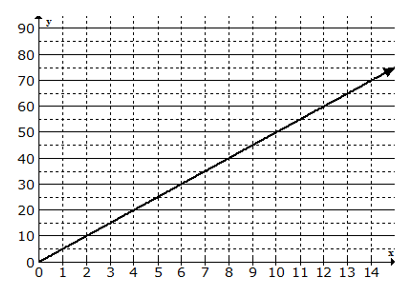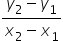Mathematics
Easy

Question

# Given the graph, find the equation of the line in point slope form.## y - 20 = ½ (x - 4)y - 20 = 5(x - 4)y + 20= 5(x + 4)y - 20 = 1(x - 4)Hint:

## The correct answer is: y - 20 = 5(x - 4)

### Taking point (0 , 0 ) and (4,20)Step 1 : slope is == 20-0/4-0 = 5From the graph m is 5Step 2 :  point is (4,20)y - y1 = m (x - x1) is the point–slope form of the equation of a line.So, Substituting the valuesequation of line is y - 20 = 5(x - 4) .

First find the slope using any two clear points .
Then using one point and slope construct the equation in point slope form  .

### Related Questions to study#### With Turito Foundation.#### Get an Expert Advice From Turito.